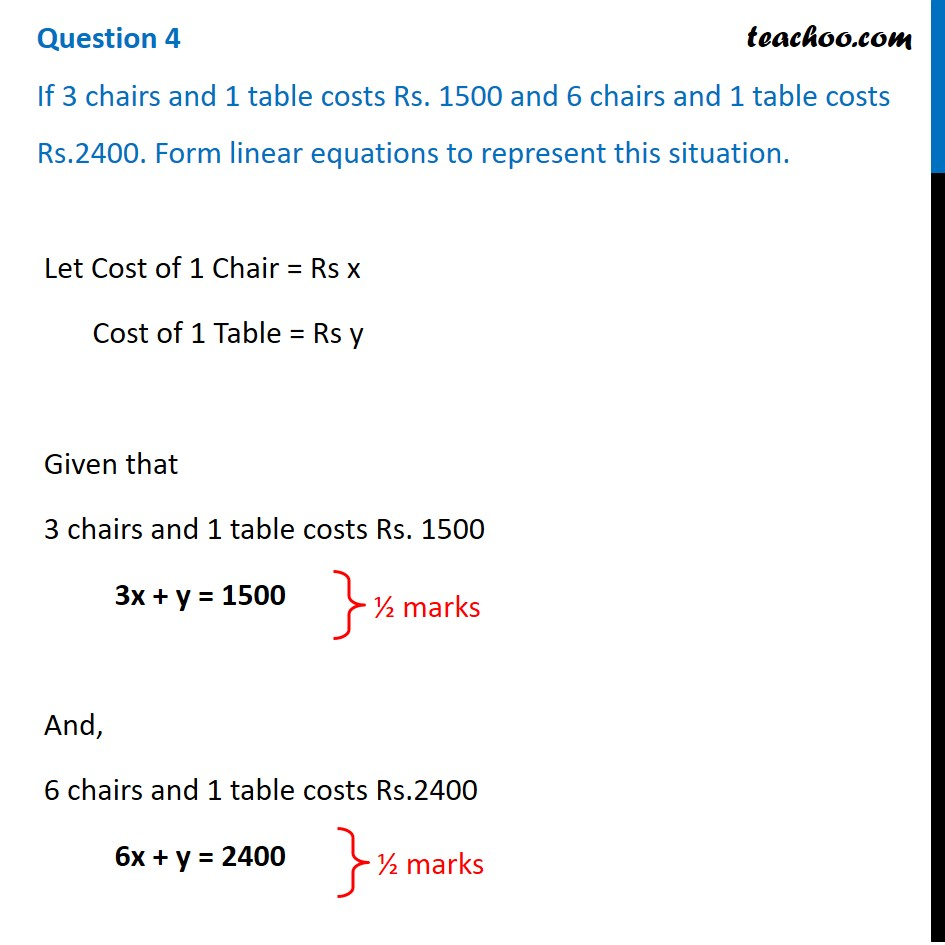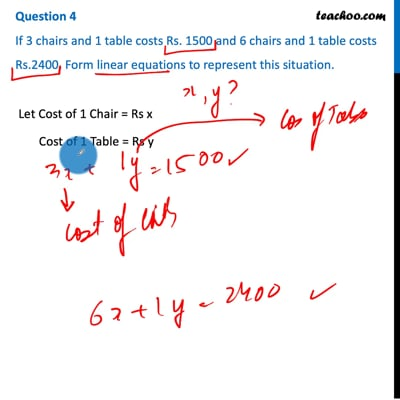CBSE Class 10 Sample Paper for 2021 Boards - Maths Standard

Class 10
Solutions of Sample Papers for Class 10 Boards

## If 3 chairs and 1 table costs Rs. 1500 and 6 chairs and 1 table costs Rs.2400. Form linear equations to represent this situation.This video is only available for Teachoo black users

### Transcript

Question 4 If 3 chairs and 1 table costs Rs. 1500 and 6 chairs and 1 table costs Rs.2400. Form linear equations to represent this situation. Let Cost of 1 Chair = Rs x Cost of 1 Table = Rs y Given that 3 chairs and 1 table costs Rs. 1500 3x + y = 1500 And, 6 chairs and 1 table costs Rs.2400 6x + y = 2400